# 2.3 序列

## 2.3.1 嵌套偶对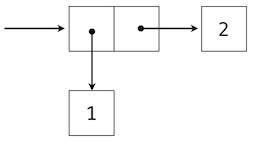``````>>> ((1, 2), (3, 4))
((1, 2), (3, 4))
``````## 2.3.2 递归列表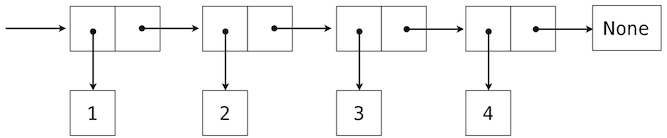``````>>> (1, (2, (3, (4, None))))
(1, (2, (3, (4, None))))
``````

• 它的第一个元素，以及
• 序列的其余部分。

``````>>> empty_rlist = None
>>> def make_rlist(first, rest):
"""Make a recursive list from its first element and the rest."""
return (first, rest)
>>> def first(s):
"""Return the first element of a recursive list s."""
return s
>>> def rest(s):
"""Return the rest of the elements of a recursive list s."""
return s
``````

• 如果一个递归列表`s`由元素`f`和列表`r`构造，那么`first(s)`返回`f`，并且`rest(s)`返回`r`

``````>>> counts = make_rlist(1, make_rlist(2, make_rlist(3, make_rlist(4, empty_rlist))))
>>> first(counts)
1
>>> rest(counts)
(2, (3, (4, None)))
``````

``````>>> def len_rlist(s):
"""Return the length of recursive list s."""
length = 0
while s != empty_rlist:
s, length = rest(s), length + 1
return length
>>> def getitem_rlist(s, i):
"""Return the element at index i of recursive list s."""
while i > 0:
s, i = rest(s), i - 1
return first(s)
``````

``````>>> len_rlist(counts)
4
>>> getitem_rlist(counts, 1)  # The second item has index 1
2
``````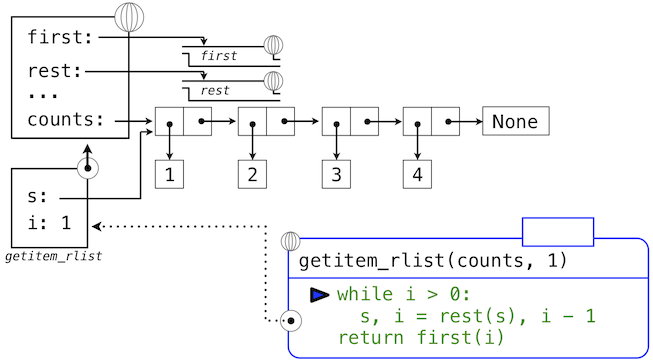`while`头部中的表达式求值为真，这会导致`while`语句组中的赋值语句被执行：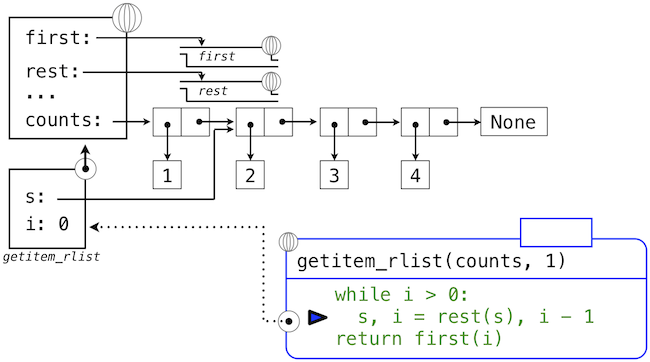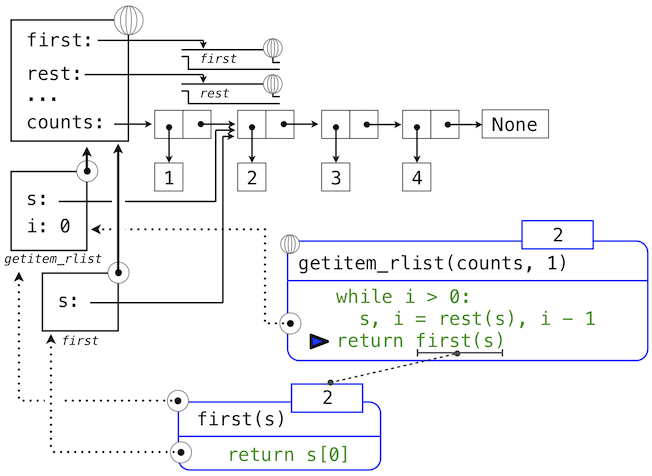## 2.3.2 元组 II

``````>>> digits = (1, 8, 2, 8)
>>> len(digits)
4
>>> digits
8
``````

``````>>> (2, 7) + digits * 2
(2, 7, 1, 8, 2, 8, 1, 8, 2, 8)
``````

``````>>> alternates = (-1, 2, -3, 4, -5)
>>> tuple(map(abs, alternates))
(1, 2, 3, 4, 5)
``````

`map`函数非常重要，因为它依赖于序列抽象：我们不需要关心底层元组的结构，只需要能够独立访问每个元素，以便将它作为参数传入用于映射的函数中（这里是`abs`）。

## 2.3.4 序列迭代

``````>>> def count(s, value):
"""Count the number of occurrences of value in sequence s."""
total, index = 0, 0
while index < len(s):
if s[index] == value:
total = total + 1
index = index + 1
>>> count(digits, 8)
2
``````

Python `for`语句可以通过直接迭代元素值来简化这个函数体，完全不需要引入`index`。例如（原文是`For example`，为双关语），我们可以写成：

``````>>> def count(s, value):
"""Count the number of occurrences of value in sequence s."""
total = 0
for elem in s:
if elem == value:
total = total + 1
>>> count(digits, 8)
2
``````

`for`语句按照以下过程来执行：

1. 求出头部表达式`<expression>`，它必须产生一个可迭代的值。
2. 对于序列中的每个元素值，按顺序：
1. 在局部环境中将变量名`<name>`绑定到这个值上。
2. 执行语句组`<suite>`

``````>>> pairs = ((1, 2), (2, 2), (2, 3), (4, 4))
``````

``````>>> for x, y in pairs:
if x == y:
same_count = same_count + 1
>>> same_count
2
``````

``````>>> range(1, 10)  # Includes 1, but not 10
range(1, 10)
``````

``````>>> tuple(range(5, 8))
(5, 6, 7)
``````

``````>>> total = 0
>>> for k in range(5, 8):
total = total + k
>>> total
18
``````

``````>>> for _ in range(3):
print('Go Bears!')

Go Bears!
Go Bears!
Go Bears!
``````

## 2.3.5 序列抽象

``````>>> digits
(1, 8, 2, 8)
>>> 2 in digits
True
>>> 1828 not in digits
True
``````

Python 中，序列切片的表示类似于元素选择，使用方括号。冒号分割了起始和结束下标。任何边界上的省略都被当作极限值：起始下标为 0，结束下标是序列长度。

``````>>> digits[0:2]
(1, 8)
>>> digits[1:]
(8, 2, 8)
``````

Python 序列抽象的这些额外行为的枚举，给我们了一个机会来反思数据抽象通常由什么构成。抽象的丰富性（也就是说它包含行为的多少）非常重要。对于使用抽象的用户，额外的行为很有帮助，另一方面，满足新类型抽象的丰富需求是个挑战。为了确保我们的递归列表实现支持这些额外的行为，需要一些工作量。另一个抽象丰富性的负面结果是，它们需要用户长时间学习。

## 2.3.6 字符串

``````>>> 'I am string!'
'I am string!'
>>> "I've got an apostrophe"
"I've got an apostrophe"
>>> '您好'
'您好'
``````

``````>>> city = 'Berkeley'
>>> len(city)
8
>>> city
'k'
``````

``````>>> city = 'Berkeley'
>>> len(city)
8
>>> city
'k'
``````

``````>>> 'here' in "Where's Waldo?"
True
``````

``````>>> 'Mississippi'.count('i')
4
>>> 'Mississippi'.count('issi')
1
``````

``````>>> """The Zen of Python
``````

``````>>> str(2) + ' is an element of ' + str(digits)
'2 is an element of (1, 8, 2, 8)'
``````

`str`函数可以以任何类型的参数调用，并返回合适的值，这个机制是后面的泛用函数的主题。

``````>>> '1234'.isnumeric()
True
>>> 'rOBERT dE nIRO'.swapcase()
'Robert De Niro'
>>> 'snakeyes'.upper().endswith('YES')
True
``````

## 2.3.7 接口约定

1. 对前`n`个斐波那契数中的偶数求和。
2. 列出一个名称中的所有缩写字母，它包含每个大写单词的首字母。

`````` enumerate     map    filter  accumulate
-----------    ---    ------  ----------
naturals(n)    fib    iseven     sum
``````

``````>>> def fib(k):
"""Compute the kth Fibonacci number."""
prev, curr = 1, 0  # curr is the first Fibonacci number.
for _ in range(k - 1):
prev, curr = curr, prev + curr
return curr
``````

``````>>> def iseven(n):
return n % 2 == 0
``````

`map``filter`函数是序列操作，我们已经见过了`map`，它在序列中的每个元素上调用函数并且收集结果。`filter`函数接受序列，并且返回序列中谓词为真的元素。两个函数都返回间接对象，`map``filter`对象，它们是可以转换为元组或求和的可迭代对象。

``````>>> nums = (5, 6, -7, -8, 9)
>>> tuple(filter(iseven, nums))
(6, -8)
>>> sum(map(abs, nums))
35
``````

``````>>> def sum_even_fibs(n):
"""Sum the first n even Fibonacci numbers."""
return sum(filter(iseven, map(fib, range(1, n+1))))
>>> sum_even_fibs(20)
3382
``````

``````enumerate  filter   map   accumulate
---------  ------  -----  ----------
words    iscap   first    tuple
``````

``````>>> tuple('Spaces between words'.split())
('Spaces', 'between', 'words')
``````

``````>>> def first(s):
return s
>>> def iscap(s):
return len(s) > 0 and s.isupper()
``````

``````>>> def acronym(name):
"""Return a tuple of the letters that form the acronym for name."""
return tuple(map(first, filter(iscap, name.split())))
>>> acronym('University of California Berkeley Undergraduate Graphics Group')
('U', 'C', 'B', 'U', 'G', 'G')
``````

``````<map expression> for <name> in <sequence expression> if <filter expression>
``````

``````>>> def acronym(name):
return tuple(w for w in name.split() if iscap(w))
>>> def sum_even_fibs(n):
return sum(fib(k) for k in range(1, n+1) if fib(k) % 2 == 0)
``````

``````>>> from operator import mul
>>> from functools import reduce
>>> reduce(mul, (1, 2, 3, 4, 5))
120
``````

``````>>> def product_even_fibs(n):
"""Return the product of the first n even Fibonacci numbers, except 0."""
return reduce(mul, filter(iseven, map(fib, range(2, n+1))))
>>> product_even_fibs(20)
123476336640
``````

`map``filter``reduce`对应的高阶过程的组合会再一次在第四章出现，在我们思考多台计算机之间的分布式计算方法的时候。